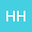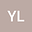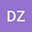On the ground states for the X-ray free electron lasers Schrödinger equation
•••Yuan Li
Central China Normal University
Author ProfileDun Zhao
Lanzhou University
Author Profile## Abstract

We consider the following X-ray free electron lasers Schr\”{o}dinger equation \begin{equation*} (i\nabla-A)^2u+V(x)u-\frac{\mu}{|x|} u=\left(\frac{1}{|x|}*|u|^2\right) u-K(x)|u|^{q-2} u, \,\, x\in \mathbb{R}^3, \end{equation*} where $A\in L_{loc}^2(\mathbb{R}^3,\mathbb{R}^3)$ denotes the magnetic potential such that the magnetic field $B=\text{curl} \, A$ is $\mathbb{Z}^{3}$-periodic, $\mu\in \mathbb{R}$, $K \in L^{\infty}\left(\mathbb{R}^3\right)$ is $\mathbb{Z}^{3}$ -periodic and non-negative, $q\in(2,4)$. Using the variational method, based on a profile decomposition of the Cerami sequence in $H^1_A\left(\mathbb{R}^3\right)$, we obtain the existence of the ground state solution for suitable $\mu\geq0$. When $\mu<0$ is small, we also obtain the non-existence. Furthermore, we give a description for the asymptotic behaviour of the ground states as $\mu \to 0^+$.
06 Apr 2022Submitted to Mathematical Methods in the Applied Sciences
07 Apr 2022Submission Checks Completed
07 Apr 2022Assigned to Editor
12 Apr 2022Reviewer(s) Assigned
23 Jul 2022Review(s) Completed, Editorial Evaluation Pending
24 Aug 2022Editorial Decision: Revise Major